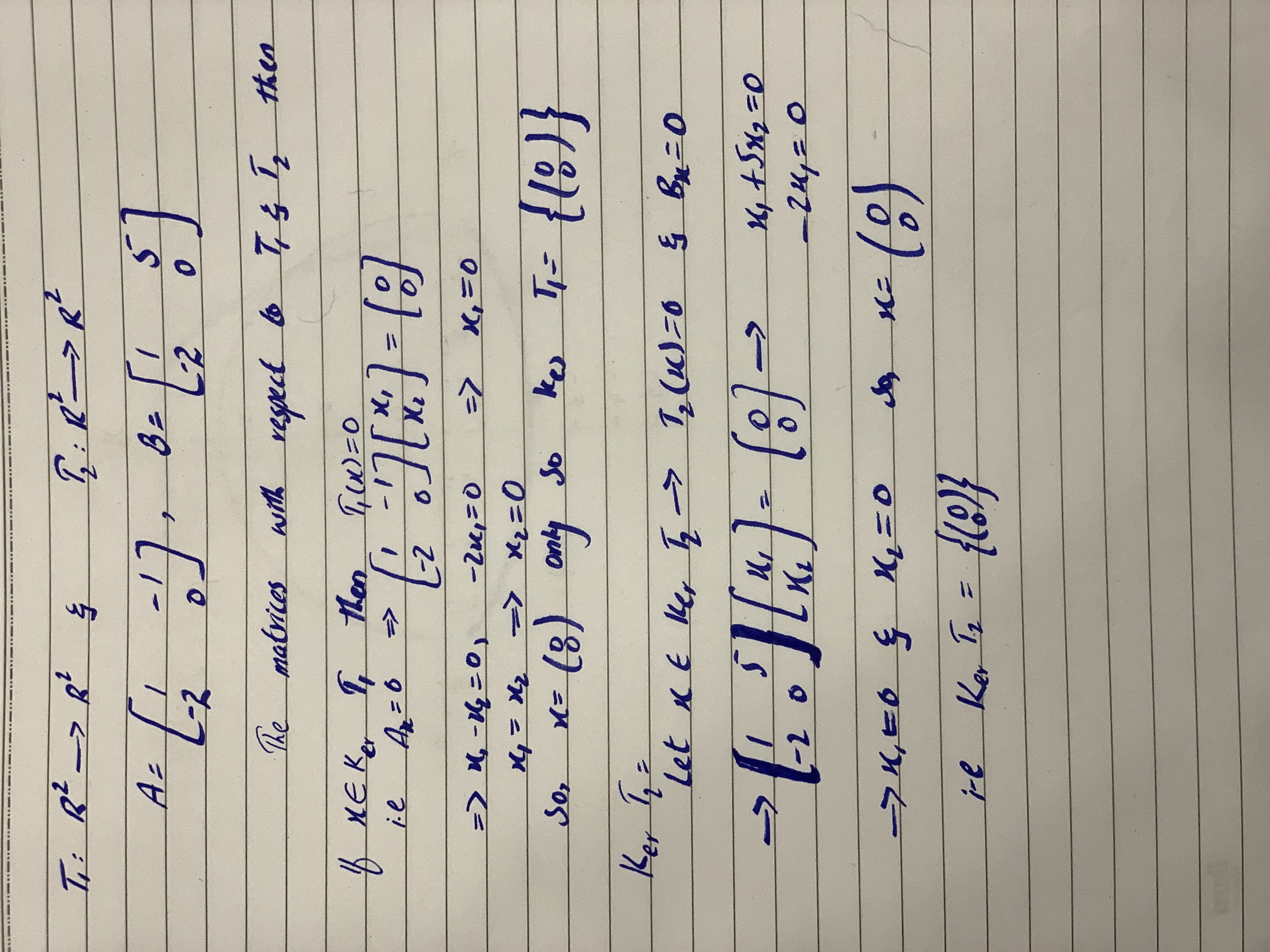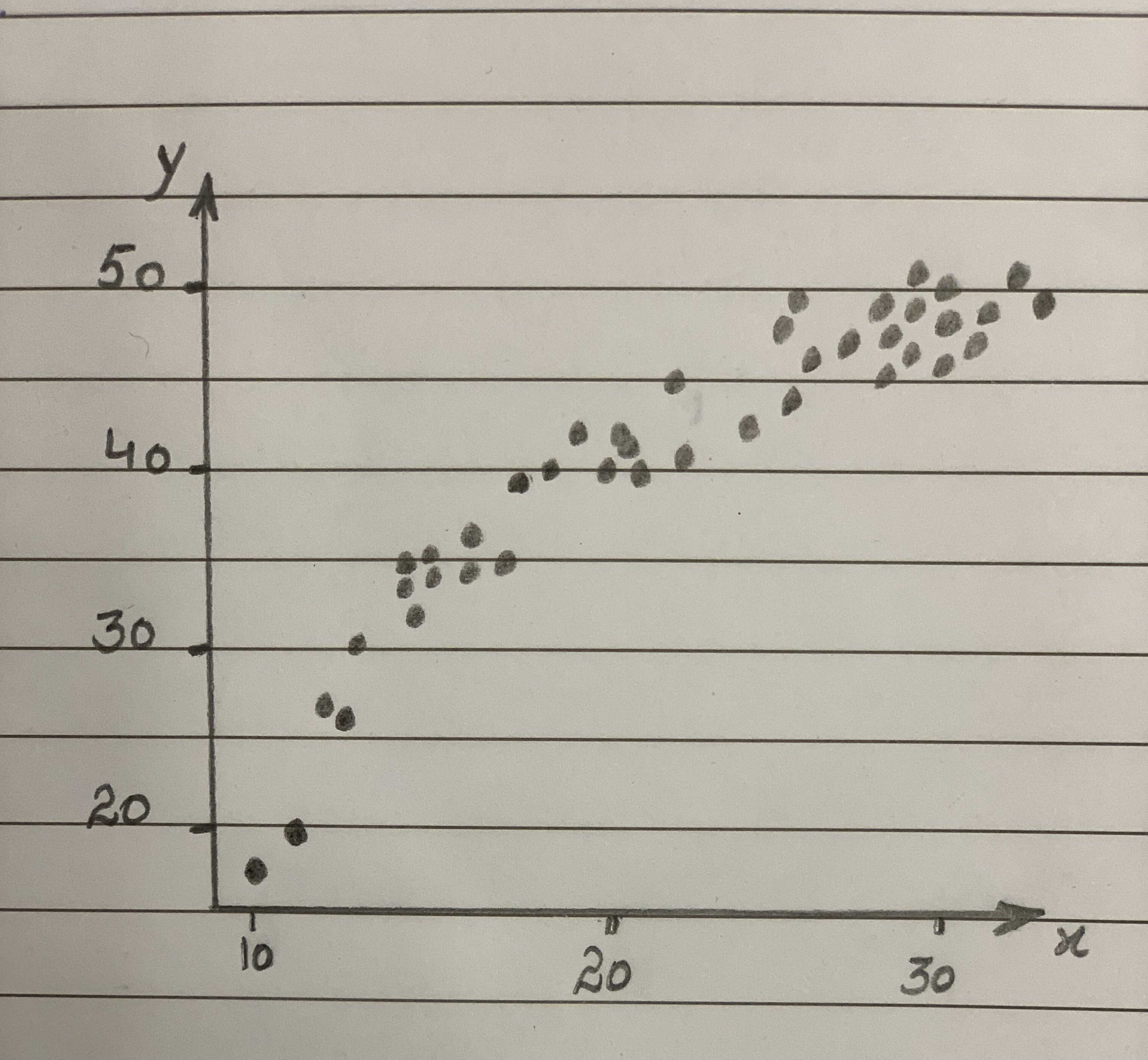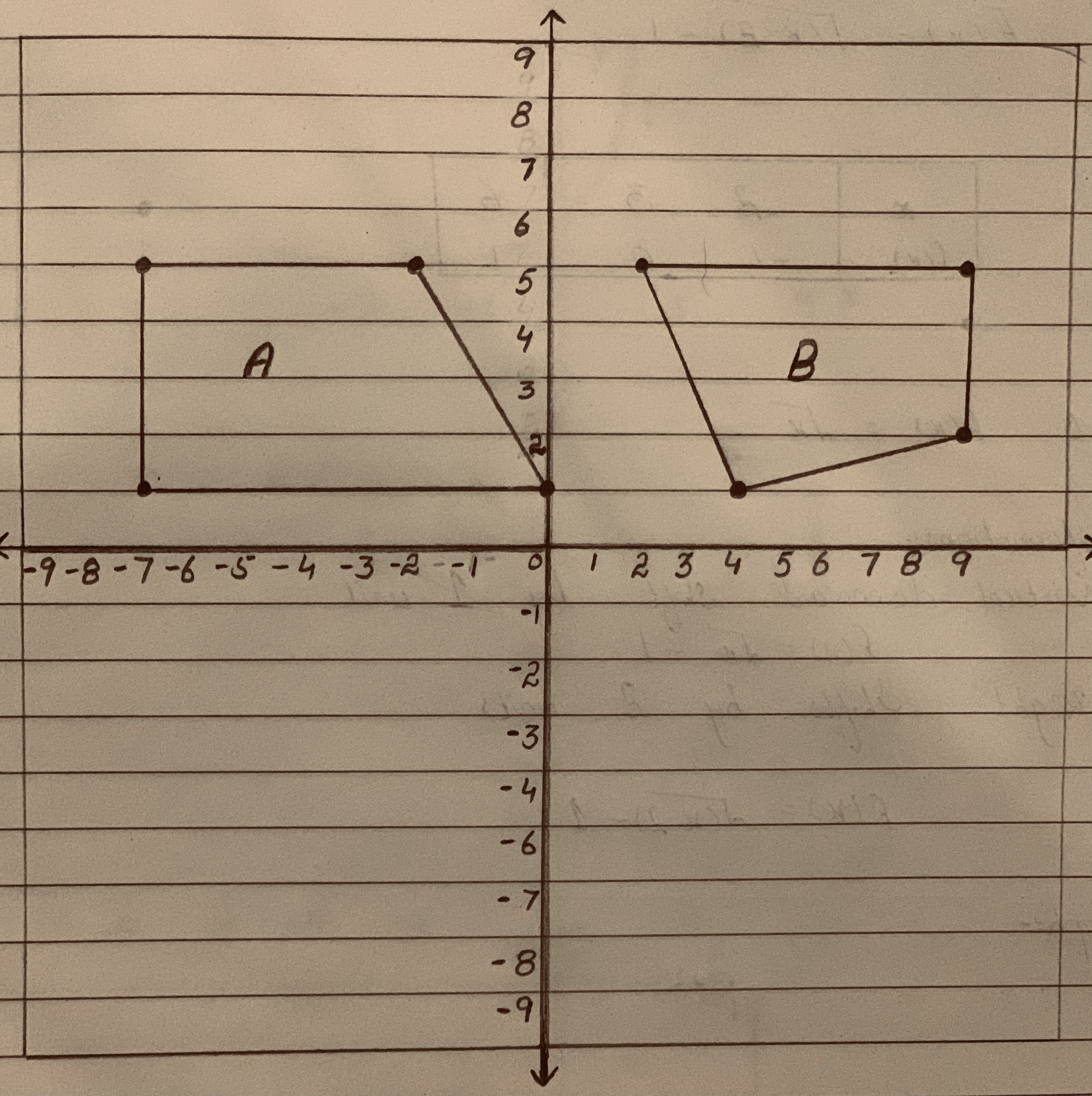# In this problem, allow T1: RR2rightarrowRR2 and T2: RR2rightarrowR2 be linear transformations. Find Ker(T_1), Ker(T_2), Ker(T_3) of the respective matrices A=[(1,-1),(-2,0)],B=[(1,5),(-2,0)]Question
Performing transformationsIn this problem, allow $$T1: RR2rightarrowRR2 and T2: RR2rightarrowR2$$ be linear transformations. Find $$Ker(T_1), Ker(T_2), Ker(T_3)$$ of the respective matrices
$$A=[(1,-1),(-2,0)],B=[(1,5),(-2,0)]$$2020-10-28### Relevant QuestionsIn this problem, allow $$T_1: \mathbb{R}^2 \rightarrow \mathbb{R}^2$$ and $$T_2: \mathbb{R}^2 \rightarrow \mathbb{R}^2$$ be linear transformations. Find Ker(T_1), Ker(T_2), Ker(T_3) of the respective matrices:
$$A=\begin{bmatrix}1 & -1 \\-2 & 0 \end{bmatrix} , B=\begin{bmatrix}1 & 5 \\-2 & 0 \end{bmatrix}$$Let T $$\displaystyle{P}_{{2}}\rightarrow\mathbb{R}^{{3}}$$ be a transformation given by
$$\displaystyle{T}{\left({f{{\left({x}\right)}}}\right)}={\left[\begin{array}{c} {f{{\left({0}\right)}}}\\{f{{\left({1}\right)}}}\\{2}{f{{\left({1}\right)}}}\end{array}\right]}$$
(a)Then show that T is a linear transformation.
(b)Find and describe the kernel(null space) of T i.e Ker(T) and range of T.
(c)Show that T is one-to-one.An object of mass M is held in place by an applied force Fand A pulley system as shown in Figure. The pulleys aremassless and frictionless. Find (a) the tension in each section of rope, $$\displaystyle{T}_{{1}},{T}_{{2}},{T}_{{3}},{T}_{{4}}$$ and $$\displaystyle{T}_{{5}}$$ and b) the magnitude of F. Suggestion: Draw afree-body diagram for each pulley.Determine the transformations of the function f(x) to get the function $$g(x) =1/2f(5(x−3))−12$$ (The transformations happen in c, b, a, d order)Your friend attempted to describe the transformations applied to the graph of $$y=sinx$$ to give the equation $$f(x)=1/2sin(-1/3(x+30))+1$$.
They think the following transformations have been applied. Which transformations have been identified correctly, and which have not? Justify your answer.
a) f(x) has been reflected vertically.
b) f(x) has been stretched vertically by a factor of 2.
c) f(x) has been stretched horizontally by a factor of 3.
d) f(x) has a phase shift left 30 degrees.
e) f(x) has been translated up 1 unit.Consider the scatterplot of y versus x in Output B.6 above.
a. Which power transformations on y should be considered to straighten the scatterplot?
b. Which power transformations on x should be considered to straighten the scatterplot?List a sequence of transformations, in words, to convert the graph of $$f(x)=x^2$$ into the graph of $$g(x)=-6(x-2)^2+5$$.Lily wants to define a transformation (or series of transformations) using only rotations, reflections or translations that takes Figure A to Figure B.Which statement about the transformation that Lily wants to define is true?
A. It can be defined with two reflections.
B.It can be defined with one rotation and one translation.
C. It cannot be defined because Figure A and Figure B are not congruent.
D.It cannot be defined because the longest side of Figure B is on the bottom.